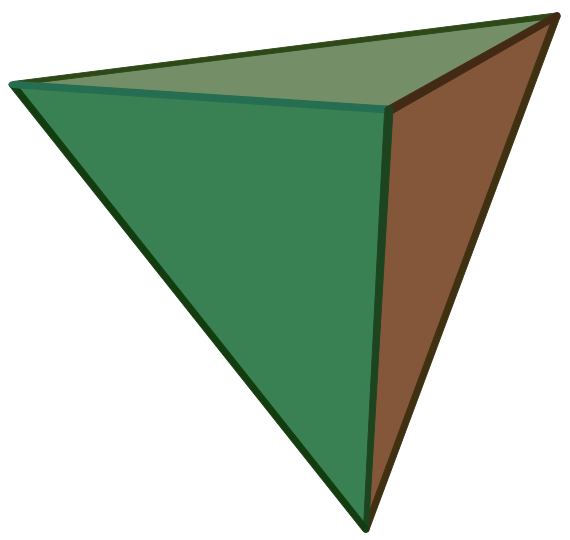# How Many Faces, Edges, And Vertices Does A Triangular Pyramid Have?

4 faces
thanked the writer.
4 faces, 6 edges and 6 vertices
thanked the writer.
A triangular pyramid has 4 faces
thanked the writer.
The answer is 4. And yes the base is a face
thanked the writer.
8 edges
thanked the writer.
4 faces
thanked the writer.
A triangular pyramid has 4 faces, 4 vertices and 6 edges

Rest of you are wrong. You suck!
thanked the writer.
The same number it had here.

The picture shows a view of a triangular pyramid from above. All of the edges (6) and vertices (4) are shown, and all but one of the faces. The bottom face is out of view. There are 4 faces total.thanked the writer.
It has 3 faces, you are all wrong, 1 base and 2 on top
thanked the writer.
4 thats so easy
thanked the writer.
F=5      E=8     V=5
thanked the writer.
12
thanked the writer.
8
thanked the writer.
5 or 4
thanked the writer.
There is only 5 faces
thanked the writer.
12
thanked the writer.
Edges
thanked the writer.
The base is counted as a face (there is 1), as are each of the triangular sides whose base is a side of the base (there are 3). 1 + 3 = 4.
thanked the writer.
It normally has five flat surfaces if you include the bottom of the pyramid, but if you draw one with three vertex's it only has 4 flat surfaces including the bottom.  Hope that makes sense.
thanked the writer.
A triangular pyramid has 4 flat surfaces,  3 vertical and 1 horizontal.   The horizontal flat
surface is the pyramid's floor.   Seesee
thanked the writer.
6 faces
thanked the writer.
4 Faces 6 vertices 6 edges
thanked the writer.
I dunn0 I need it 4 homework!!
thanked the writer.
Edges =6
vertices =4
faces =4
thanked the writer.
4 faces 4 vertices 9 edges
thanked the writer.
Its got 4
thanked the writer.
4 hello do yall people know that lolz jk but it's 4 so easy
thanked the writer.
There are four. The base is the first, and the other three are the triangles that connect to the sides of the base
thanked the writer.
This website (Blurt it) is stupid I can't get anything done with it all the people that see this your just waisting your time so look on another website I'm saying this cause I care
thanked the writer.
It is 4 I needed it for my math homework and my teacher said it was 4 faces
thanked the writer.
I know for a fact that there are 9 and only 9 edges in a triangular prism got it dorks kiss kiss
thanked the writer.
4 faces the bottom counts as a base for any one else who needed to know
thanked the writer.